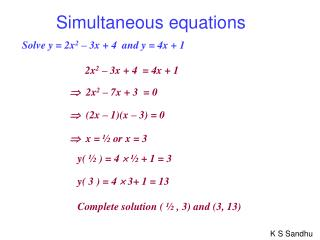DownloadDownload PresentationSimultaneous equations

# Simultaneous equations

Télécharger la présentation## Simultaneous equations

- - - - - - - - - - - - - - - - - - - - - - - - - - - E N D - - - - - - - - - - - - - - - - - - - - - - - - - - -
##### Presentation Transcript

1. Simultaneous equations Solve y = 2x2 – 3x + 4 and y = 4x + 1 2x2 – 3x + 4 = 4x + 1  2x2 – 7x + 3 = 0  (2x – 1)(x – 3) = 0  x = ½ or x = 3 y( ½ ) = 4  ½ + 1 = 3 y( 3 ) = 4  3+ 1 = 13 Complete solution ( ½ , 3) and (3, 13)

2. Simultaneous equations Solve y = x2 + 3x - 18 and y = 2x + 2 x2 + 3x - 18 = 2x + 2  x2 + x - 20 = 0  (x + 5)(x - 4) = 0  x = -5 or x = 4 y( -5 ) = 2 (-5) + 2 = -8 y( 4 ) = 2  4+ 2 = 10 Complete solution ( -5 , -8) and (4, 10)

3. Simultaneous equations Show that the simultaneous equations y = x2 + 3x - 18 and y = 2x – 20 have no real solution. x2 + 3x - 18 = 2x - 20  x2 + x + 2 = 0 a =1, b = 1 and c = 2  b2 – 4ac = 1 – 4  1  2 = - 7 < 0 The line and the curve do not intersect i.e. no real solutions

4. Simultaneous equations The graph of y = 2x + k meets the graph of y = x2 + 3x – 18 at only one point. Find the value of k. x2 + 3x - 18 = 2x + k  x2 + x - k - 18 = 0 a =1, b = 1 and c = -k - 18  b2 – 4ac = 1 – 4  1  (- k – 18) = 0  1 + 4k + 72 = 0  k = -73/4

5. Simultaneous equations It is given that x and y satisfy the simultaneous equations: y – x = 4 and 2x2 + xy + y2 = 8 (i) Show that x2 + 3x + 2 = 0 (ii) Solve the equations. (i) y – x = 4  y = x + 4  2x2 + xy + y2 = 8  2x2 + x(x + 4) + (x + 4)2 = 8  2x2 + x2 + 4x + x2 + 8x + 16 = 8  4x2 + 12x + 8 = 0  x2 + 3x + 2 = 0 (ii)  (x + 2)(x + 1)= 0  x = - 2 or x = - 1 Complete solution (-2, 2) or (-1, 3)

6. Simultaneous equations Solve the simultaneous equations. x + y = 1 and x2 - xy + y2 = 7 x + y = 1  y = 1 - x  x2 – x(1 – x) + (1 – x)2 = 7  x2 – x + x2 + 1 – 2x + x2 = 7  3x2 - 3x - 6 = 0  x2 - x - 2 = 0  (x + 1)(x - 2)= 0  x = - 1 or x = 2 Complete solution (-1, 2) or (2, -1)

7. Solve the simultaneous equationsx + y =2 (1) x2 + 2y2 = 11 (2)  Substitute for x from (1) into (2) (2 – y)2 + 2y2 = 11 4 – 4y + y2 + 2y2 = 11 3y2– 4y– 7 = 0  Factorise and solve for y (3y – 7)(y + 1) = 0  Substitute for y in (1)

8. Solve the simultaneous equations y = x2 (1) x + y = 6 (2)  Substitute for x from (2) into (1) y = (6 – y)2 y = 36 – 12y + y2 y2– 13y+ 36 = 0  Factorise and solve for y (y – 4)(y - 9) = 0 y = 4 or y = 9  Substitute for x in (2)